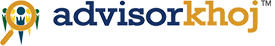# How Compound interest works

## Personal Finance

by | Comments | 252 Downloaded | 16474 viewed | | | 4.0 | 21 votes | Rate this ArticleCompound interest is the eighth wonder of the world. He who understands it, earns it... he who doesn't... pays it. - Albert Einstein

In our previous article we discussed the benefits of start investing early for wealth creation and we also discussed how it is possible using 'power of compounding'. Now, let’s understand how Compound Interest works in your favour?

Compound interest is the concept of adding earned or accumulated interest back to the principal amount, so that interest is earned on top of interest from that period onwards. The act of adding declared interest to be principal is called compounding.

It is similar to simple interest (in simple interest, the interest portion in not added to the principal) but, here the interest will be added to the principal after a certain unit of time (e.g. Monthly, Quarterly, Half – yearly and Annually etc.). Example – a savings of Rs.10,000 at 10% annual interest will become 11,000 after 12 months. Here, the interest amount is Rs.1,000. But, the interest amount after 24 months would be Rs.1,100 as at the end of 24 month the Interest is calculated on Rs.11,000 (i.e. Rs.10,000 + Rs. 1,000 – Interest earned in the 1st Year)! This is possible as the interest will be paid both on the principal and on the interest that has been added to it. In other words, we can say, interest itself earns interest!

The compounding period and the frequency of compounding apart from the interest rate are the major components of compound interest calculation. The frequency (Annual, Half-year, Quarter or month) after which an interest earned during that period added to the principal is called as compounding period. Now, let’s understand these with formulas on different compound periods.

When the interest is compounded annually then the following formula will be used to calculate the yearly compounding interest –

When the interest is compounded half - yearly then the following formula will be used to calculate the half-yearly compounding interest –

When the interest is compounded quarterly then the following formula will be used to calculate the Quarterly compounding interest –

When the interest is compounded monthly then the following formula will be used to calculate the monthly compounding interest –

In the above formula the P denotes the Principal amount. R denotes the percentage of interest and n denotes the time in years or months (n means annual, 2n means half-year, 4n means quarter and 12n means monthly).

Now, let’s understand 'how to calculate and get the results in excel'? The following shows the result of Rs.10,000 invested at 10% interest for 5 years at different compounding periods.

## Result of monthly compounding

Hope, you find that above useful. Further reading on compound interest - Wikipedia

### Pradip Chakrabarty

Pradip is a first generation entrepreneur who has built a very successful business for distribution of financial products over last 19 yrs. He is also the Founder and CEO of www.advisorkhoj.com. Pradip is well respected in the Financial Services industry and brings deep domain knowledge and leadership skills and is considered as one of the top Investment Advisors in India. He can be contacted at pradip@advisorkhoj.com

comments powered by Disqus
Search

### TOP ARTICLES

May 12, 2014

© 2016 Advisorkhoj - A Gamechanger Business Services (I) Pvt. Ltd. Brand - All Rights Reserved